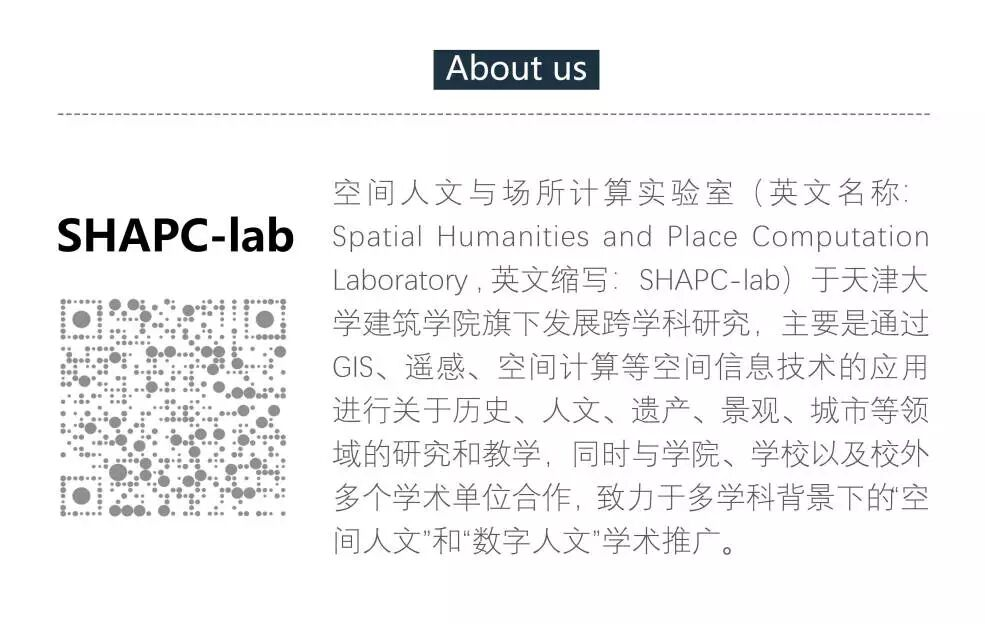# 疫情词云|基于大数据和自然语言处理的舆情热点分析新年伊始，新型冠状病毒（2019-nCoV）肆虐中华，让人倍感心痛。在持续关注疫情的同时，我也不禁思考，怎样才能利用自己的专业知识为疫情贡献一点力量。在浏览有关新型冠状病毒的新闻时，一些关键词不断涌现：口罩、疑似病例、小汤山、驰援武汉、消毒等等，辟谣与反辟谣的新闻屡见不鲜。持续关注疫情资讯的我不禁对舆情的关注热点产生了好奇，在疫情大规模爆发的当下，主流媒体的报道究竟关注哪些热点信息？报道的重点是否有变化？出现最多的关键词是哪些？作为吃瓜群众的我们究竟怎样才能明辨是非，尽到不信谣不传谣的责任？

带着这些疑问，我通过python的网络爬虫和自然语言处理技术，对CCTV央视网的2020年1月27号的新闻进行提取分析，得到了下面的“疫情词云”图。希望通过这种方式窥探舆情热点的重心和变化趋势，从数据维度理解新闻。PART1 数据分析Y = 213.310856X^(- 0.56776567)。由方程可知，24号的方程曲率更缓，系数更小，说明24日的报道频次和关键词密度低于1月27日，可见自24日以来政府的重视程度在加大，媒体的报道热点更集中PART2 Python实战``1.  #-*- coding:utf-8 -*-  ``2.  import requests  ``3.  import re  ``4.  from bs4 import BeautifulSoup  ````1.  urllst = []  ``2.  titlelst = []  ``3.  out2 = open('新闻.txt','a',encoding='utf-8')  ``4.  with open (r'E:pyguanzhuangbingducctvCCTV新闻.txt','r',encoding='utf-8') as f:  ``5.      text = f.readlines()  ``6.      for line in text:  ``7.          title = re.findall('"title":"(.+?)"',line)  ``8.          url = re.findall(r'http://(.+?).shtml"',line)  ``9.          if url:  ``10.              urllst.append(url)  ``11.      #print(urllst)  ``12.    ``13.      for i in range(len(urllst)):  ``14.          for w in urllst[i]:  ``15.              url = 'http://'+ w + '.shtml'  ``16.              print(url)  ``17.              titlelst.append(url)  ````1.  #-*- coding:utf-8 -*-  ``2.  import requests  ``3.  import re  ``4.  from bs4 import BeautifulSoup  ``5.    ``6.  urllst = []  ``7.  titlelst = []  ``8.  out2 = open('新闻.txt','a',encoding='utf-8')  ``9.  with open (r'E:pyguanzhuangbingducctvCCTV新闻.txt','r',encoding='utf-8') as f:  ``10.      text = f.readlines()  ``11.      for line in text:  ``12.          title = re.findall('"title":"(.+?)"',line)  ``13.          url = re.findall(r'http://(.+?).shtml"',line)  ``14.          if url:  ``15.              urllst.append(url)  ``16.      #print(urllst)  ``17.    ``18.      for i in range(len(urllst)):  ``19.          for w in urllst[i]:  ``20.              url = 'http://'+ w + '.shtml'  ``21.              #print(url)  ``22.              titlelst.append(url)  ``23.      for urll in titlelst:  ``24.          #print(urll)  ``25.          try:  ``26.              r=requests.get(urll)  ``27.              r.encoding = r.apparent_encoding  ``28.              r.raise_for_status  ``29.              print(r.text)  ``30.              soup = BeautifulSoup(r.text,'lxml')  ``31.              div = soup.find_all('div',class_="content_area")  ``32.              soup2 = BeautifulSoup(str(div),'lxml')  ``33.              p = soup2.find_all('p')  ``34.              contents = re.findall('[^<p></p>]',str(p))  ``35.              print(''.join(contents))  ``36.              print(''.join(contents),file = out2 )  ``37.          except:  ``38.              print('失败')``

``1.  # -*- coding: utf-8 -*-  ``2.  import jieba  ``3.  import jieba.posseg as pseg  ``4.  # 创建停用词list  ``5.  def stopwordslist(filepath):  ``6.      stopwords = [line.strip() for line in open(filepath, 'r', encoding='utf-8').readlines()]  ``7.      return stopwords  ``8.  # 对句子进行分词  ``9.  def seg_sentence(sentence):  ``10.      jieba.load_userdict('字典.txt')   ``11.      #jieba.analyse.set_stop_words('changan_stopwords.txt')  ``12.      sentence_seged =pseg.cut(sentence.strip())  ``13.      sentence_seged = jieba.cut(sentence.strip(),cut_all=False)  ``14.      stopwords = stopwordslist('最全中文停用词.txt')  # 这里加载停用词的路径  ``15.      outstr = ''  ``16.      for word in sentence_seged:  ``17.          if word not in stopwords:  ``18.              if word != 't':  ``19.                  outstr += word  ``20.                  outstr += " "  ``21.      return outstr  ``22.  inputs = open('新闻.txt', 'r', encoding='utf-8') # 这里加载分词后文本的路径  ``23.  outputs = open('新闻_分词去停用词.txt', 'w',encoding='utf-8')  # 这里加载输出文本的路径  ``24.  for line in inputs:  ``25.      line_seg = seg_sentence(line)  # 这里的返回值是字符串  ``26.      outputs.write(line_seg + 'n')  ``27.  outputs.close()  ``28.  inputs.close()  ``29.  print('success')````1.  # -*- coding: utf-8 -*-  ``2.  #!/usr/bin/env python  ``3.  import collections  ``4.  from PIL import Image  ``5.  import numpy as np  ``6.  import matplotlib.pyplot as plt  ``7.  from scipy.misc import imread  ``8.  from wordcloud import WordCloud,ImageColorGenerator  ``9.  import random  ````1.  def random_color_func(word=None, font_size=None, position=None,  orientation=None, font_path=None, random_state=None):  ``2.      h = random.randint(330,360)  ``3.      s = int(100.0 * float(random.randint(40, 200)) / 255.0)  ``4.      l = int(100.0 * float(random.randint(20, 150)) / 255.0)  ``5.      return "hsl({}, {}%, {}%)".format(h, s, l)````1.  mask= imread('中国地图.png')  ``2.  wc = WordCloud(font_path=font_path2,  # 设置字体                ``3.                 background_color=None, mode="RGBA",  # 背景颜色  ``4.                 max_words=500,  # 词云显示的最大词数  ``5.                 mask=mask,  # 设置遮罩  ``6.                 max_font_size=130 ,   # 最大字号``7.                 random_state=40,  ``8.                 font_step= 1,  ``9.                 min_font_size=16,  ``10.                 collocations=False,  ``11.                 color_func = random_color_func)   ``12.  wc.generate(text)``13.  wc.to_file("temp.png")``

``1.  # -*- coding: utf-8 -*-  ``2.  #!/usr/bin/env python  ``3.  import collections  ``4.  from os import path  ``5.  from PIL import Image  ``6.  import numpy as np  ``7.  import matplotlib.pyplot as plt  ``8.  from scipy.misc import imread  ``9.  from wordcloud import WordCloud,ImageColorGenerator  ``10.  import random  ``11.  d = path.dirname(__file__)  ``12.  font_path2='121.TTF'  ``13.  # 读取整个文本.  ``14.  text = open('新闻处理_分词去停用词.txt','r',encoding= 'utf-8').read()  ``15.    ``16.  def random_color_func(word=None, font_size=None, position=None,  orientation=None, font_path=None, random_state=None):  ``17.      h  = random.randint(330,360)  ``18.      s = int(100.0 * float(random.randint(40, 200)) / 255.0)  ``19.      l = int(100.0 * float(random.randint(20, 150)) / 255.0)  ``20.      return "hsl({}, {}%, {}%)".format(h, s, l)  ``21.    ``22.  mask= imread('中国地图2.png')  ``23.  wc = WordCloud(font_path=font_path2,  # 设置字体                ``24.                 background_color=None, mode="RGBA",  # 背景颜色  ``25.                 max_words=500,  # 词云显示的最大词数  ``26.                 mask=mask,  # 设置背景图片  ``27.                 max_font_size=130 ,  ``28.                 random_state=40,  ``29.                 font_step= 1,  ``30.                 min_font_size=16,  ``31.                 collocations=False,  ``32.                 color_func = random_color_func)   ``33.  # 生成词云  ``34.  wc.generate(text)  ``35.  wc.to_file("temp.png")  ``36.  print (wc.words_)  ``37.  print(wc.layout_)  ``38.    ``39.  plt.figure()  ``40.  plt.imshow(wc, interpolation='bilinear')  ``41.  plt.axis("off")  ``42.  plt.show()``PART3 小结

1

2

Fiddler抓包，并保存抓包下来的全部会话

3

4

5

6

撰稿/肖天意